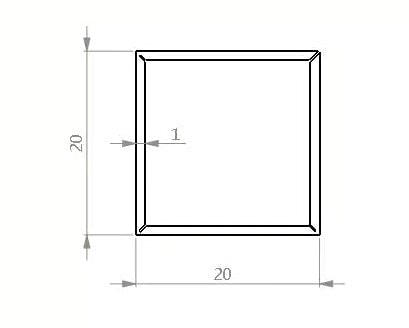## Grooving Machine For Sale

The metal sheet grooving machine is often used in the building decoration industry. It can conduct V-shaped slotting for metal sheets such as stainless steel sheet and aluminum plate, so as to minimize the arc radius of the edge of the workpiece after bending, reduce the bending force required for bending sheet materials, and pre position the bending edge length before bending, so as to ensure the accuracy of the size of the bending edge length.

### Horizontal Grooving Machine

The horizontal type V grooving machine has much higher efficiency when slotting the metal sheet.

### Vertical V Grooving Machine

For the slotting of irregular sheet metal, vertical V grooving machine is the best choice.

### Four-side Grooving Machine

The new generation of designed structure enables the machine to groove on four sides of the same plate within the specified size.

# How To Calculate The Unfolded Length Of Groove Bending?

Calculation of the difference between the unfolded length of grooved bending and non-grooved bendingGrooved bending

For grooved bending, the total length is calculated as：

L=20-0.4+20-2X0.4+20-0.4=58.4

Non-grooved bending

For non-grooved bending, the total length is calculated as：

L=(20-1xt+k)+(20-2xt+2xk)+(20-1xt+1xk)=(20-1+0.2)+(20-2+2×0.2)+(20-1+0.2)=56.8mm

Note: t is the material thickness and k is the coefficient.

The size of k is related to the material thickness, generally between 0.2 and 0.25. The thicker the material, the larger the k value.

In this case, k is chosen as 0.2.

## 1. Square tube grooving

As shown in the figure, now to use the groove and bend a 20 × 20mm square tube, the thickness of the plate is 1mm.The remaining material thickness a of the groove is related to the size of the customer’s right-angle arc after bending, and also to the size of the material thickness t.

Generally speaking, when the material thickness is less than or equal to 1mm, a=0.4mm; when the material thickness is greater than 1mm, a=t/2.

The unfolded length of the illustrated example is:

L=2×(L1+L2)=2x((Edge length-1xa-0.2)+(Edge length-2xa))=2x((20-1×0.4-0.2)+(20-2×0.4))=77.2mm

Note: One end of L1 is grooved off, regardless of the thickness of the material, are deducted 0.2mm

## 2. Z-shape grooving

The following combination of a Z-shaped grooved and ungrooved is used to calculate the bending unfolding length.For 2mm plate, the remaining material thickness of the groove is taken t/2=1mm, (if the customer requires a smaller direct arc, a value should be chosen less than 1mm)

So the unfolding length.

L=15-1+20-2×1+15-1=46mm

For a 2mm thick plate, without groove bending, the factor k is chosen to be 0.25, so the unfolded length

L=(15-2+0.25)+(20-2×2+2×0.25)+(15-2+0.25)=43mm

## 3. Multi-bend grooving

The following calculation is presented with an example of a multi-bend workpiece grooved and ungrooved.For a 3mm thick plate, assuming that the customer requires a small rounding of the right angle, the remaining material thickness is chosen to be 0.5mm.

Unfolded length:

L=(40-0.5)+(30-2×0.5)+(30-2×0.5)+(10-0.5)=107mm；

For a 3mm thick plate, the coefficient k is chosen to be 0.25, so the unfolding length:

L=(40-3+0.25)+(30-6+2×0.25)+(30-6+2×0.25)+(10-3+0.25)=93.5mm

## 4. Reverse bending after grooving

The method of calculating the unfolding is presented below through an example of reverse bending after grooving.For the reverse bend after grooving as shown in the figure, the length of the unfolding is calculated as

L=(20-2+0.2)+(30-2×2+2×0.2)+(20-2+0.2)=62.8mm

Need help?

If you want to know the price of our machine, or have any questions, pls feel free to contact us.

error: Content is protected !!
Scroll to Top